# Stem and Leaf Diagrams

GCSEKS3Level 1-3AQACambridge iGCSEEdexcel

## Stem and Leaf Diagrams

A stem and leaf diagram is a way of displaying a collection of numbers. The ‘stem’ consists of the first part of every number, usually the first digit(s) and the ‘leaf’ consists of the latter part of every number, usually the last digit. We need to know how to draw them and how to use them.

Make sure you are happy with the following topics before continuing.

Level 1-3GCSEKS3AQAEdexcelCambridge iGCSE

## Drawing a Stem and Leaf Diagram

We need to be able to draw stem and leaf diagrams, along with a key that tells us what the numbers in the diagram mean.

Example:  Scott measured the height of all the people in his choir. The values, in cm, are given below.

$154,\,\,180,\,\,176,\,\,153,$ $\, 162,\,\,165,\,\,154,\,\,186,$

$190,\,\,187,\,\,176,\,\,176,$ $\, 172,\,\,182,\,\,177,\,\,169$

Draw a stem-and-leaf diagram to represent Scott’s data.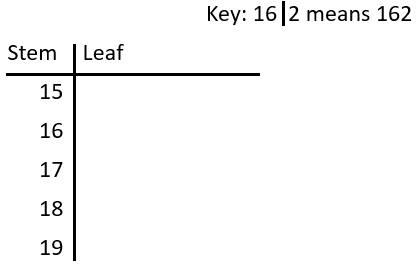Step 1: Figure out what our key will be. The stem will be the first two digits, whilst the leaf will be the last digit. Our key is any number that is in the list, represented in terms of a stem and leaf.

Step 2: Use our key to draw our stem part of the diagram, which will have to include: $15, \, 16, \, 17, \, 18, \, 19$ as shown.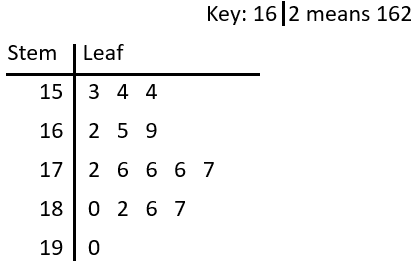Step 3: Rewrite the data in numerical order.

$153,\,\,154,\,\,154,\,\,162,$ $\,165,\,\,169,\,\,172,\,\,176,$

$176,\,\,176,\,\,177,\,\,180,$ $\,182,\,\,186,\,\,187,\,\,190$

Step 4: Fill in the ‘leaf’ values (the last digit of every value) alongside the correct ‘stem’ value, to complete the stem and leaf diagram.

Level 1-3GCSEKS3AQAEdexcelCambridge iGCSE

## Using a Stem and Leaf Diagram

We also need to be able to interpret stem and leaf diagrams to be able to solve questions on the mean, median, mode and range of numbers.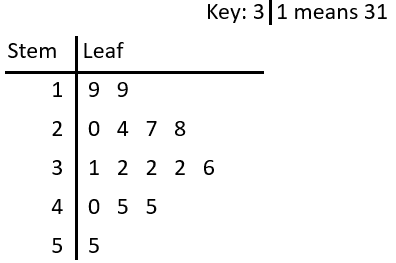Example:  Ramona collected the ages of colleagues and recorded her results using a stem and leaf diagram.

Find the mode, median and range of her colleagues ages.

Mode means the number that appears most often. Here we can see that the number $2$ appears three times in the ‘$3$’ row – more times than any other value appears, therefore the mode is $32$.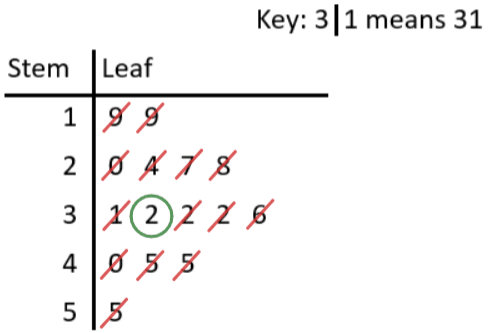Median is the middle value. A common method for finding the median is to cross out the smallest value, then the largest, then the $2$nd smallest, then the $2$nd largest, and so on until you only have $1$ value left. This is shown in the diagram below. Therefore the median is $32$.

Range is the largest value minus the smallest. Reading off the diagram, we see the smallest value is $19$, whilst the largest is $55$. So, we get

$\text{range }=55-19=36$

Level 1-3GCSEKS3AQAEdexcelCambridge iGCSE

## Stem and Leaf Diagrams Example Questions

All of these values are $2$-digit numbers, so the first digits will be in the ‘stem’ section, and the second digits will be in the ‘leaf’ section. Since the lowest number begins with a ‘$1$‘ and the highest begins with a ‘$4$‘, our stem and leaf diagram, including the key, should look like the one below: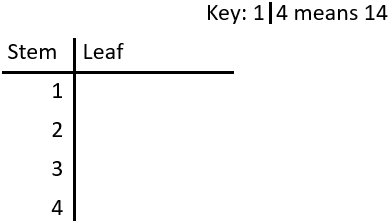(Any value can be chosen as an example for the key.)

To ensure that you don’t inadvertently omit any of the values, it would be a good idea to write them out in order (check that your list contains the same number of values as in the question, so in this example, check that you have $12$ values):

$14,\,\,19,\,\,22,\,\,24,\,\,29,\,\,32,$ $\,35,\,\,35,\,\,36,\,\,38,\,\,41,\,\,47$

To complete the stem and leaf diagram itself, first of all look at all the values that start with $1$ ($14$ and $19$), and enter their last digits in the leaf section of the $1$ stem (so you would write ‘$4$‘ and ‘$9$‘ in the leaf section).  Then repeat this process values beginning with $2$, then with values beginning with $3$ etc.

Your completed stem and leaf diagram should look like the below: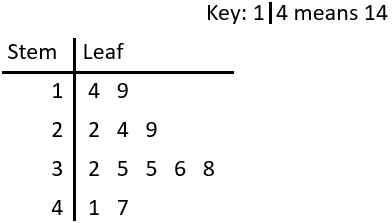Gold Standard Education

a) The only number to appear more than once is the ‘$2$’ leaf on the ‘$1$’ part of the stem. Therefore, the mode is $12$.

b) The largest value is $51$ (stem of ‘$5$’ and leaf of ‘$1$’ ) and the smallest is $9$ (stem of ‘$0$‘ and leaf of ‘$9$’). So, the range is

$51-9=42$

c) All the journeys under $20$ minutes are the ones that appear in the ‘$0$’ stem or the ‘$1$’ stem. From the diagram, we can see that there are $8$ journeys in these two sections, and in total there are $15$ times recorded.  This means that $8$ out of $15$ journeys were under $20$ minutes, which can be written as the following fraction:

$\dfrac{8}{15}$

To convert a fraction to a percentage, we need to divide the top of the fraction by the bottom and multiply by $100$:

$\dfrac{8}{15}\times100 = 53.3\%$Gold Standard Education

a)  The range is the difference between the weight of the heaviest calf and the weight of the lightest calf.  The heaviest calf will be in the ‘$14$’ stem.  There is only one calf in this stem and its leaf is $3$, so the weight of the heaviest calf is $143$ pounds.  The lightest calf will be the calf with the lowest value  in the ‘$9$’ stem, so the weight of the lightest calf is $93$ pounds.  Therefore the range of the calves’ weights is

$143-93=50\text{ pounds}$

b)  The modal weight is the most frequently occurring weight.  In the ‘$12$’ stem, we can see that there are $3$ values of $1$, more than any other in the stem and leaf diagram.  A value of $1$ in the ‘$12$’ stem represents a weight of $121$ pounds.

c)  The mean weight of the calves is the total weight divided by the total number of calves.  By counting the number of digits in the leaf section, we can see that there are $24$ calves in total.  The sum of their weights can be calculated as follows:

$93+97+97+98+101+103+106+106+109+112+112+114+114+115+118+120+121+121+121+123+126+132+138+143=2740\text{ pounds}$

(Since there are a lot of numbers to add up, I would perform the above calculation twice to ensure you arrive at the same answer.  It might even be safer to work out the subtotals for each stem section and then add up these subtotals.)

The mean weight of the calves can be calculated as follows:

$\dfrac{2740\text{ pounds}}{24\text{ calves}} = 114\text{ pounds}$

d)  The mean weight of the $24$ calves is $114$ pounds. Introducing another calf to the data set that is greater than $114$ pounds will increase the mean weight of the calves.Gold Standard Education

a)  The median is the middle value.  By counting up the number of values in the leaf section, we can see that there is a total of $17$ values.  To work out which value gives us the median, we need to remember the following formula:

$\dfrac{n+1}{2}$ (where $n$ represents the total number of values)

Since there are $17$ values in total, $n = 17$, so:

$\dfrac{17+1}{2} = 9$

The median comes from the $9$th value (do not make the mistake of thinking that the median is $9$ points).  Count the values in the leaf sections row by row until you reach the $9$th value (so in the ‘$0$’ stem, $2$ is value $1$, $4$ is value $2$, $7$ is value $3$ and in the ‘$1$’ stem, $0$ is value $4$ etc.).  If you have counted correctly, then the $9$th has a stem of ‘$1$’ and a leaf of ‘$9$‘, meaning that the median number of points scored is $19$.

b)  Jamal has played in $17$ matches in total.  In these matches, he has scored $10$ points or less on $4$ occasions (don’t forget to include the value $10$).  Therefore he has scored $10$ points or less in $4$ out of the $17$ matches, which can be expressed as the following fraction:

$\dfrac{4}{17}$

c)  In the $17$ matches that Jamal plays, he scores between $20$ and $35$ points on $5$ occasions.  This can be expressed as the following fraction:

$\dfrac{5}{17}$

The question wants us to give the answer as a percentage rather than as a fraction.  To convert a fraction to a percentage, divide the top by the bottom and multiply by $100$:

$\dfrac{5}{17}\times 100 = 29\%$

d)  First of all, we need to work the number of matches in which Jamal has scored over $25$ points.  Jamal has scored more than $25$ points on $7$ out of $17$ occasions.  Since the team always wins when Jamal scores over $25$ points (and loses when he doesn’t), then the team wins $7$ times out of $17$.  Therefore the probability of winning the next game can be expressed as the following fraction:

$\dfrac{7}{17}$

This fraction is the probability of the team winning the next game, but we are asked to give the answer as a decimal.  To convert a fraction to a decimal, simply divide the top by the bottom:

$7\div17=0.4$Gold Standard Education

a)  The modal score for Spanish is the most frequently occurring score for Spanish.  In the stem of $5$, there are $2$ values of $7$, more than other since there are no other duplicate scores for Spanish, so the modal score is $57$.

b)  The modal group for French is the group (not score) which has the highest number of values in it.  Make sure to look at the values on the left of the stem column, and not the Spanish values which are to the right.  In the French side of the stem and leaf diagram, there are $5$ values listed in the $7$ stem group.  The modal group is therefore the $70$s or the $70$$79$ group, since values that belong to this group must begin with a $7$.

c)  The range is the difference between the highest and the lowest value.

The highest score for French is $87$ (stem of $8$, leaf of $7$) and the lowest is $42$ (stem of $4$, leaf of $2$).  Therefore the range for French is $87-42=45$.

The highest value for Spanish is $89$ (stem of $8$, leaf of $9$) and the lowest is $32$ (stem of $3$, leaf of $2$).  Therefore the range for Spanish is $89-32=57$.

The question asks for the difference between the range for French and the range for Spanish which can be calculated as follows:

$57-45=12$

d)  The median is the middle score.  In order to work out which value will give us our median, we need to know how many values there are.  There are $19$ values for French and $19$ values for Spanish.  To work out the median, we need to remember the following short formula where $n$ represents the total number of values:

$\text{median}=\dfrac{n+1}{2}$

In this question the value of $n$ is $19$, so the median value can be calculated as follows:

$\dfrac{19+1}{2}=10$

The median is not simply the number $10$; it is what the $10$th value represents (which will probably be different for French and Spanish).

For Spanish the first value is $32$ (stem $3$, leaf $2$), the second $36$ (stem $3$, leaf $6$), third $39$ (stem $3$, leaf $9$), fourth $41$ (stem $4$, leaf $1$) etc.  Continuing, we will find the tenth value for Spanish which has a value of $60$.

Finding the $10$th value for French is a little bit harder due to the fact that this is a back-to-back stem and leaf diagram, so the values on the left of the stem (the French values) are in ascending order from right to left.  The first value is $42$ (stem $4$, leaf $2$), the second $46$ (stem $4$, leaf $6$), third $47$ (stem $4$, leaf $7$), fourth $49$ (stem $4$, leaf $9$), fifth $51$ (stem $5$, leaf $1$) etc.  Continuing, we will find the tenth value for French which is $65$.

The question asks by what percentage the French median score is greater or less than the Spanish median score, meaning we need to work out the difference between the median scores as a percentage of the median Spanish score.

The difference between the two median scores is $5$, so we need to work out $5$ as a percentage of the median Spanish score which is $60$:

$\dfrac{5}{60}\times100=8\%$

Since the median French score is greater and not less than the Spanish score, the median French score is $8\%$ greater than the median Spanish score.Gold Standard Education

## Stem and Leaf Diagrams Worksheet and Example Questions

### (NEW) Stem and Leaf Diagrams Exam Style Questions - MME

Level 1-3GCSENewOfficial MMEProduct